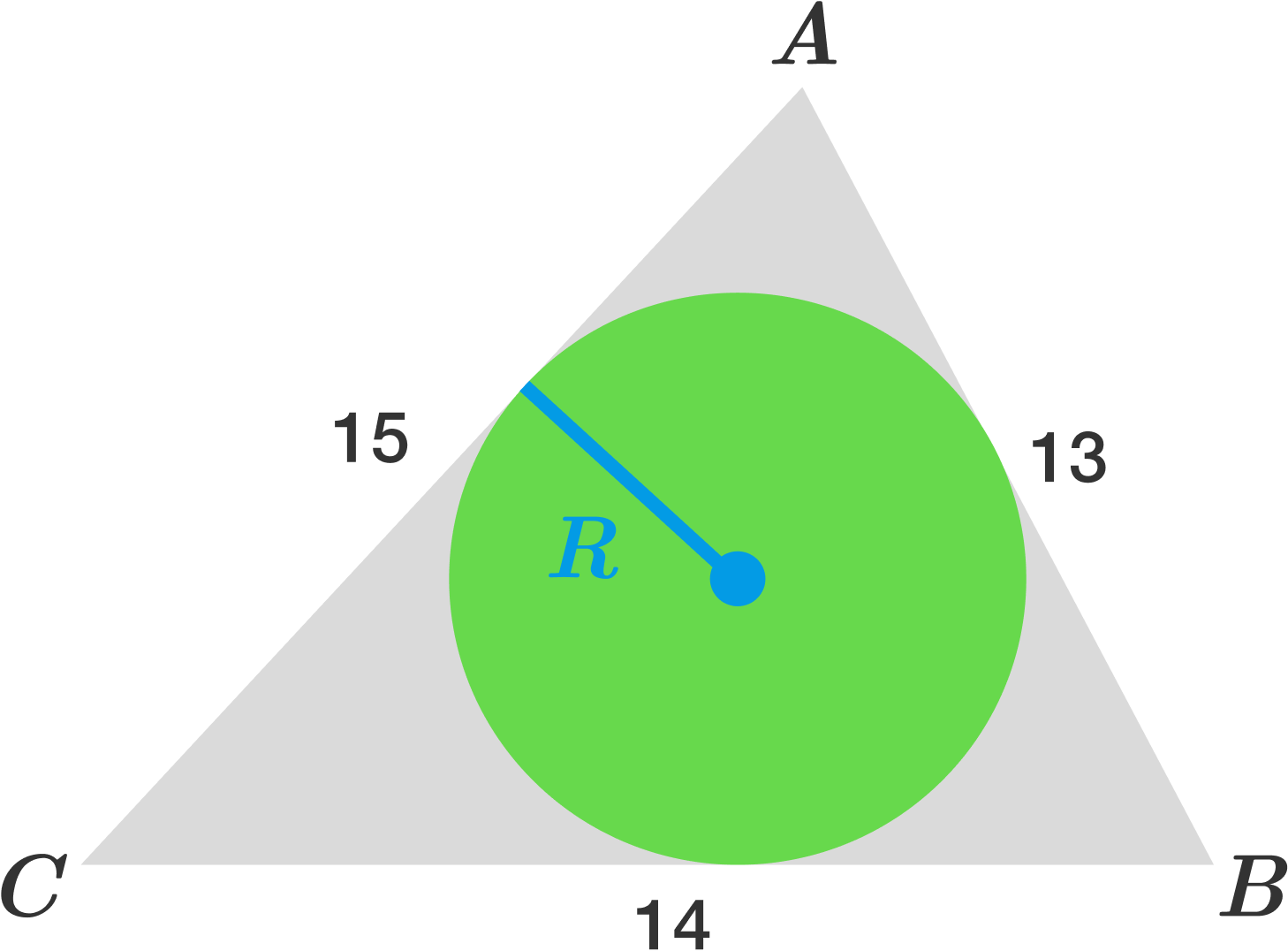Triangle $ABC$ has $AB = 13, BC = 14$, and $CA = 15$. What is the length of the inradius of $\triangle ABC$?